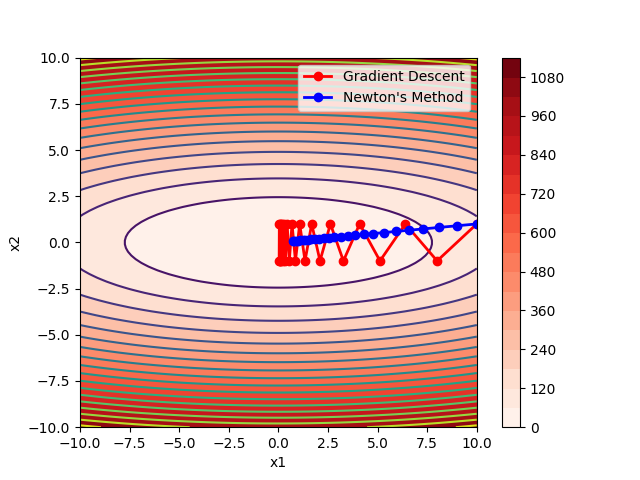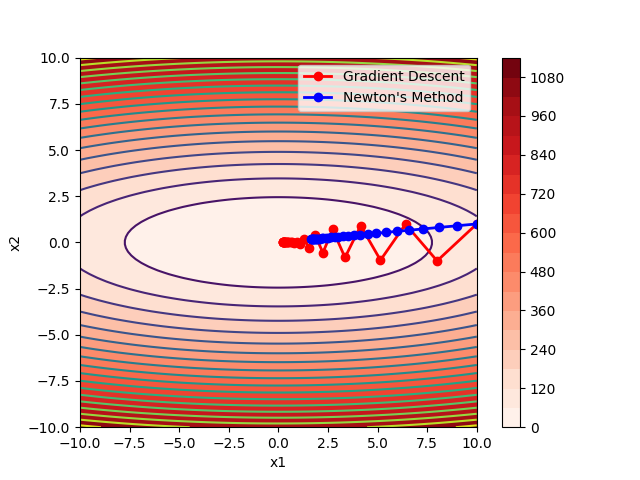# aix

2021/3/9 补充了数学层面的牛顿公式理解和在求解最小二乘问题中的应用

# Optimization

• 优化的参数
• 损失函数
• 约束（可选）

## LEAST SQUARES PROBLEMS

• 解的初始猜想
• 每一步的迭代方法
• 收敛的标准

1. 初始值$k=0$，假设初始最优解为$x_0$
2. 更新 x:
3. if $\left |\nabla J(x_{k+1}) \right | < \tau$ 停止，$x_{k+1}$为最优解，否则令$k=k+1$，重复第二步。
其中，$J(x)$ 是损失函数，$\nabla J(x_k)$是它的梯度，一般来说，梯度可以定义为一个函数的全部偏导数构成的向量（这一点与偏导数与方向导数不同，两者都为标量）。$\alpha$ 是步长，$\tau$ 是一个定义的允许的误差值。
• 梯度下降得到的是局部最优解，一个局部最小值（local minimum），而不一定是全局的最小值（global minimum）
• 梯度下降的性能和表现取决于它的步长，如果步长太大，会导致无法收敛；而小的步长会增加迭代的次数，增加开销。
• 梯度下降需要我们能够写出来损失函数和计算损失函数的梯度，有的时候我们无法找到一个合适的损失函数去评估或者无法计算其梯度。

## Newton’s Method

### 概念

1. 初始值$k=0$，假设初始最优解为$x_0$
2. 更新 x:
3. if $\left |\nabla J(x_{k+1}) \right | < \tau$ 停止，$x_{k+1}$为最优解，否则令$k=k+1$，重复第二步。
其中，$J(x)$ 是损失函数，$\nabla J(x_k)$是它的梯度，$\alpha$ 是步长，$\tau$ 是一个定义的允许的误差值。$H(x_k)$ 是 海森矩阵（Hessian Matrix），是一个多元函数的二阶偏导数构成的方阵，描述了函数的局部曲率。

• 牛顿方法是一个二阶方法，它的每次计算都要计算二阶偏导。
• 同样由于是迭代法，依旧只能找到局部最优解，也同样很依赖 $\alpha_j$ 的选择。但总体来看，牛顿方法可以用更少次数的迭代就使得结果收敛，但每一步的计算量很大。
• 沿用牛顿的方法的思路，有另外一种优化算法被称作拟牛顿法（Quasi-Newton Method），但这些方法采用梯度信息来近似海森矩阵，所以比传统的牛顿法更加有效。

### 例子：两者的比较## Nonlinear Least Squares

### 概念

• 如：f 是多项式函数，x 是 f 的系数。
• 如：z 是初始时刻在一个导热棒上温度的分布情况，y 是最终最大温度值。f 涉及模拟热传导方程来计算 y 值，x 可以被定义为在这个物体上的热扩散率。

Ctwo

2021-03-04

2021-04-24